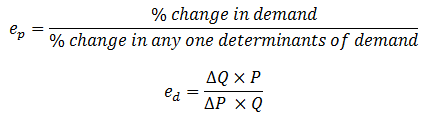# Price elasticity of demand

Price elasticity of demand (ep)

It refers the percentage change in quality demand due to the certain percentage change in price when other things remaining the same. Mathematically it is explained as:Where,
ed = elasticity of demand
∆Q = change in quantity demand
∆P = change in price commodity
P = initial price
Q = initial quantity demand